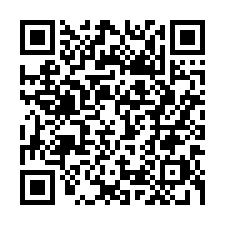# 判断一个整数的二进制第n位是否为1

0 0 0 0
0 0 1 1
0 1 0 2
0 1 1 3
1 0 0 4
1 0 1 5
1 1 0 6
1 1 1 7

## 十进制数反推权限方法一

``````// DecToBin 用于把十进制数转成2进制，使用的是除2取余法
func DecToBin(n int) string {
result := ""

if n == 0 {
return "0"
}

// 由于n是整数，所以即使n/=2(即n = n/2)是小数，最后赋值给n也只会剩下整数部分，小数部分无论多大(即使是0.9)也会丢失
for ; n > 0; n /= 2 {
lsb := n % 2
// Itoa(Int to ASCII)，但事实上就是整数转字符串,Atoi就是数字字符串转数字
result = strconv.Itoa(lsb) + result
}

return result
}
``````

``````var decimal int64 = 14
# 第一个参数必须是int64类型，所以定义的时候不能用短变量，否则它就不是int64而是int。
# 第二个参数2表示转换为二进制(同理你还可以写成8或16，分别表示转换成8进制和16进制)
binStr := strconv.FormatInt(decimal, 2)
fmt.Println(binStr)
``````

## 十进制数反推权限方法二(推荐)

### 什么是右移

``````右移两位之前: 1010

``````

### 什么是按位与

``````6:  110
2:  010
---------

``````

### 反推权限原理

``````10:  1010
1:  0001
----------

``````

``````11:  1011
1:  0001
----------

``````

### 反推权限举例

``````未移动时: 1011

``````

### 编程语言实现

``````func isOne(x int, n int) int {
return (x >> (n - 1)) & 1
}
``````

`x >> (n - 1)`表示右移n减1位，因为我们要判断第三位，就要右移两位，这样第三位就会排到第一位与二进制`1`对齐，同理，要判断第四位，就要往右移三位，这样第四位才会排到第一位，与二进制`1`对齐，所以要n减1。

`(x >> (n - 1)) & 1`整句表示x往右移动n减1位之后，再与十进制数1“按位与”，注意`&`后面那个1是十进制的1，不是二进制，因为二进制需要以`0b`开头，但是这不重要，因为十进制的1转成二进制后，它还是1，这是两个进制数相同的地方。

``````func isOne(x int, n int) int {
return x >> (n - 1) & 1
}
``````

``````func isOne(x int, n int) bool {
return (x>>(n-1))&1 == 1
}
``````

## 十进制数反推权限方法三(推荐)

``````A B C D
1 0 1 1
``````

``````A => 1000
B => 0100
C => 0010
D => 0001
``````

``````A => 0b1000
B => 0b0100
C => 0b0010
D => 0b0001
``````

``````1000 | 0010 | 0001 = 11

# 计算如下
1000
0010
0001
----
1011
``````

``````1011
0100
----
0000
``````

``````11 & 0b1000 = 8 =>(0b1000转十进制为8)
11 & 0b0010 = 2 =>(0b0010转十进制为2)
11 & 0b0001 = 1 =>(0b0001转十进制为1)
``````

``````package main

import "fmt"

const (
A int = 0b1000
B int = 0b0100
C int = 0b0010
D int = 0b0001
)

// calcFlag 用于计算并返回多个选项按位或的结果
func calcFlags(flags []int) int {
sum := 0
for _, v := range flags {
// 把所有选项做“按位或”操作
sum = sum | v
}
return sum
}

func addFlag(x int, flag int) int {
return x | flag
}

// delFlag 用于从x中删除某个flag，比如(A|B)&(^B)=A
// ^flag 表示按位取反(golang中按位取反与异或操作符共用一个)
func delFlag(x int, flag int) int {
return x & (^flag)
}

// checkFlag 用于检测某个选项是否被选中
func checkFlag(x int, flag int) bool {
return x&flag == flag
}

func main() {
// 假设我选了ACD
options := []int{A, C}
// 计算ACD做“按位与”运算后的和
sum := calcFlags(options)
// 添加D
// 删除C
sum = delFlag(sum, C)
// 这个数字用于存储到数据库
fmt.Println("sum:", sum)

// 当从数据库取出sum后，就可以用它来判断ABCD哪个选项哪个选了，哪个没选
isAChecked := checkFlag(sum, A)
isBChecked := checkFlag(sum, B)
isCChecked := checkFlag(sum, C)
isDChecked := checkFlag(sum, D)
fmt.Println("A:", isAChecked, "\nB:", isBChecked, "\nC:", isCChecked, "\nD:", isDChecked)

// === 打印结果 ===
// sum: 9
// A: true
// B: false
// C: false
// D: true

}
``````

## 十进制数反推权限方法四(推荐)

### 什么是掩码

``````原二进制码：1011

``````

``````未左移前：0001

``````

### 使用掩码获取对应位

``````1011
0100
----
0000
``````

`1011&0100 = 0`

``````1011
0010
----
0010
``````

``````A B C D
1 0 1 1
``````
``````A => 1000
B => 0100
C => 0010
D => 0001
``````

``````func isOne(x int, n int) bool {
return x&(1<<(n-1)) != 0
}
``````0 评论

0

## 扫码在手机查看iPhone请用自带相机扫安卓用UC/QQ浏览器扫